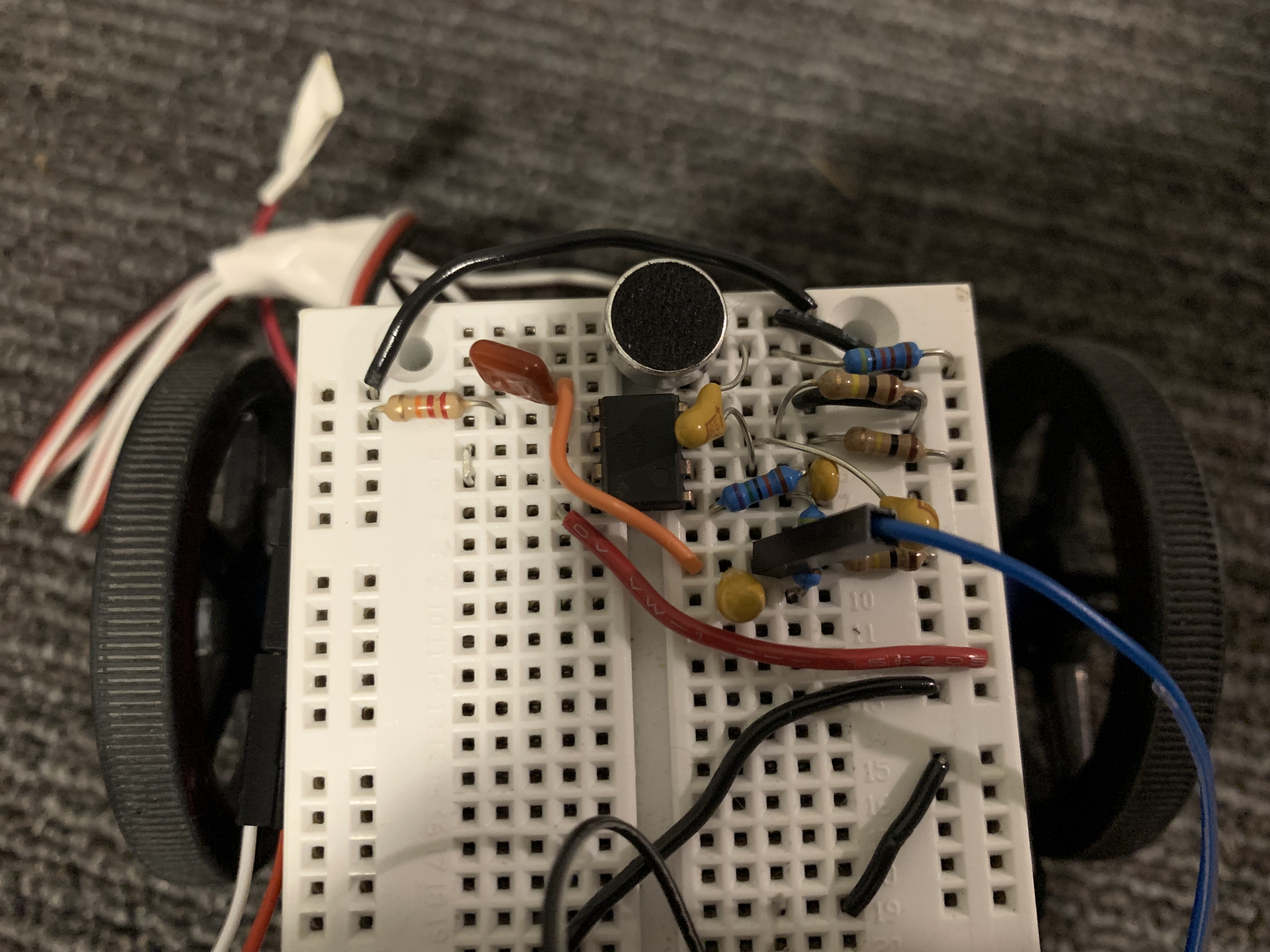### Objective

1. Build a microphone with amplifier circuit and test using Matlab
2. Use a high pass filter to generate a frequency response
3. Generate FFTs using the Arduino

### LT Spice

First, we built filters using LT Spice to see how an ideal, simulated filter frequency response ought to look before diving into our own. We used a 1.2 kOhm resistor and a 0.1 uF capacitor to simulate both a low and a high pass filter.

Figure 1: Schematic of the LT Spice Filters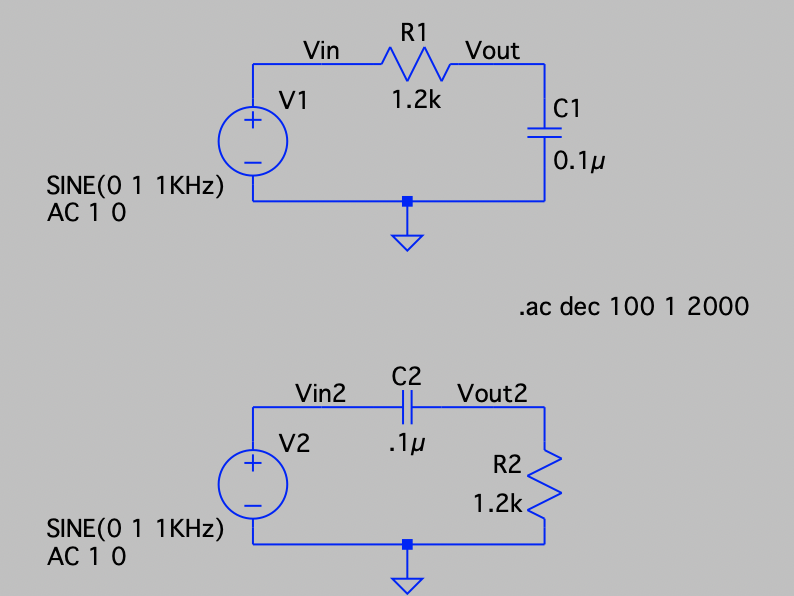By running the circuit, we could observe the entire frequency response. After zooming in to the -3dB frequency, it was found that the cutoff freqency for both the high pass and the low pass filter was 1.32 kHz.

Figures 2 and 3: Low Pass Filter with Cutoff Frequency 1.32 kHz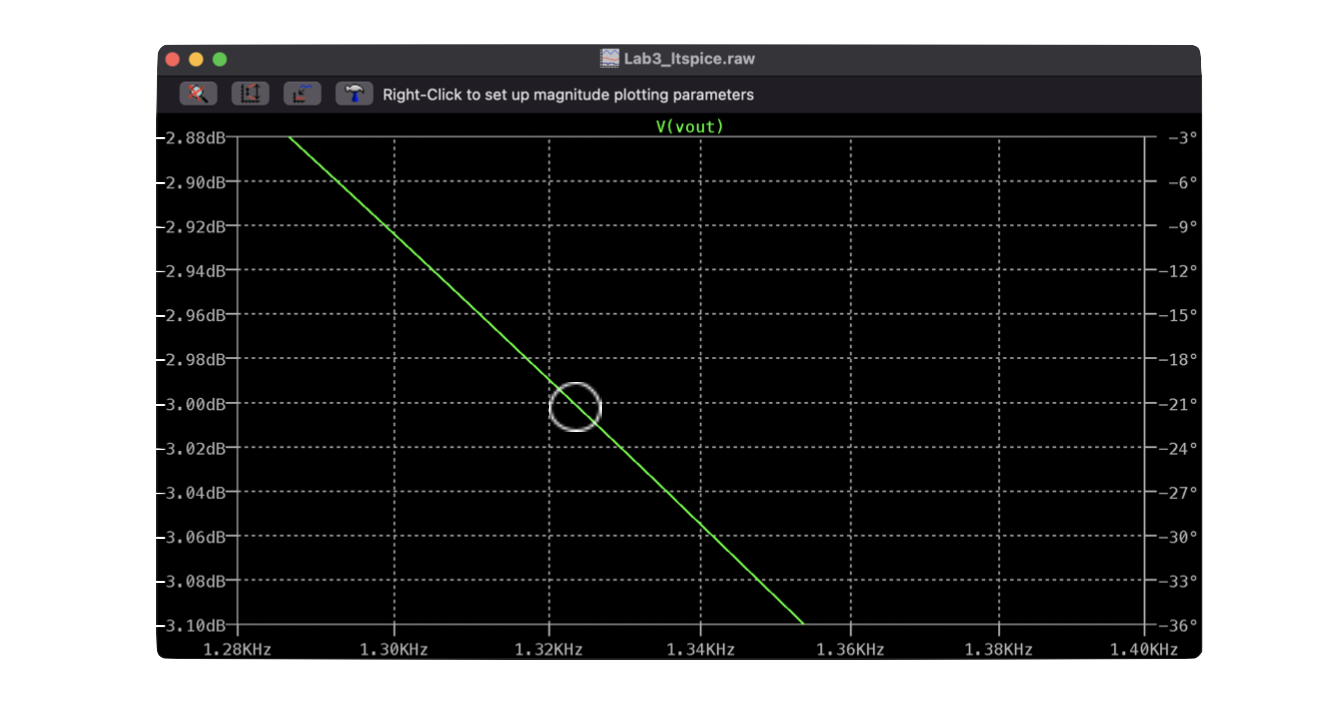### The Microphone

First, the microphone had to be breadboarded to the robot. We used pin A5 on the arduino to receive the analog signal coming out of the microphone.

Figure 4: The Microphone Schematic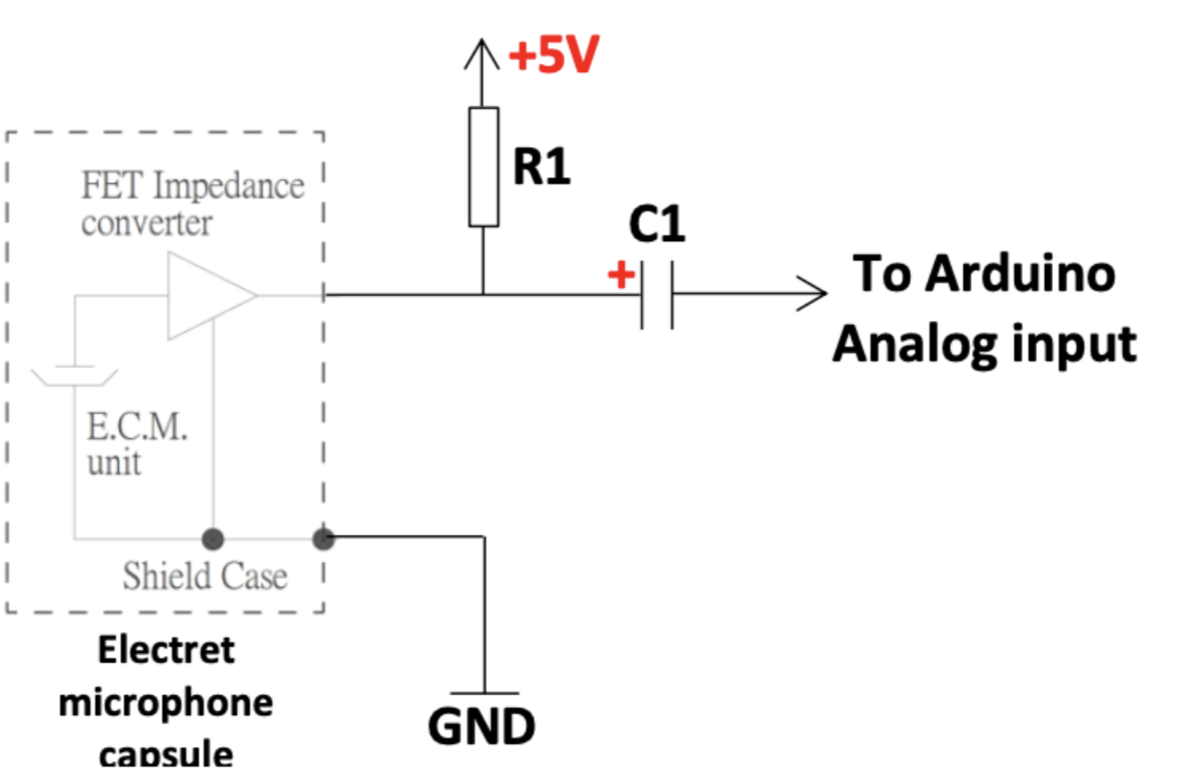Instead of doing an `AnalogRead()`, which can be slow, we set up the ATMega’s ADC to convert this microphone signal to a digital signal in free run mode. Then, the digital signal is sent over serial to MATLAB. The baud rate must be changed to 230400 so MATLAB can receive the microphone inputs and generate a graph of intensity vs freqeuncy. With everything connected and the correct serial port in MATLAB chosen, we then generate a 500 Hz signal while MATLAB “records” the Arduino output. The generated graph clearly shows a spike at around 500 Hz.

Figure 5: The Unamplified 500 Hz Response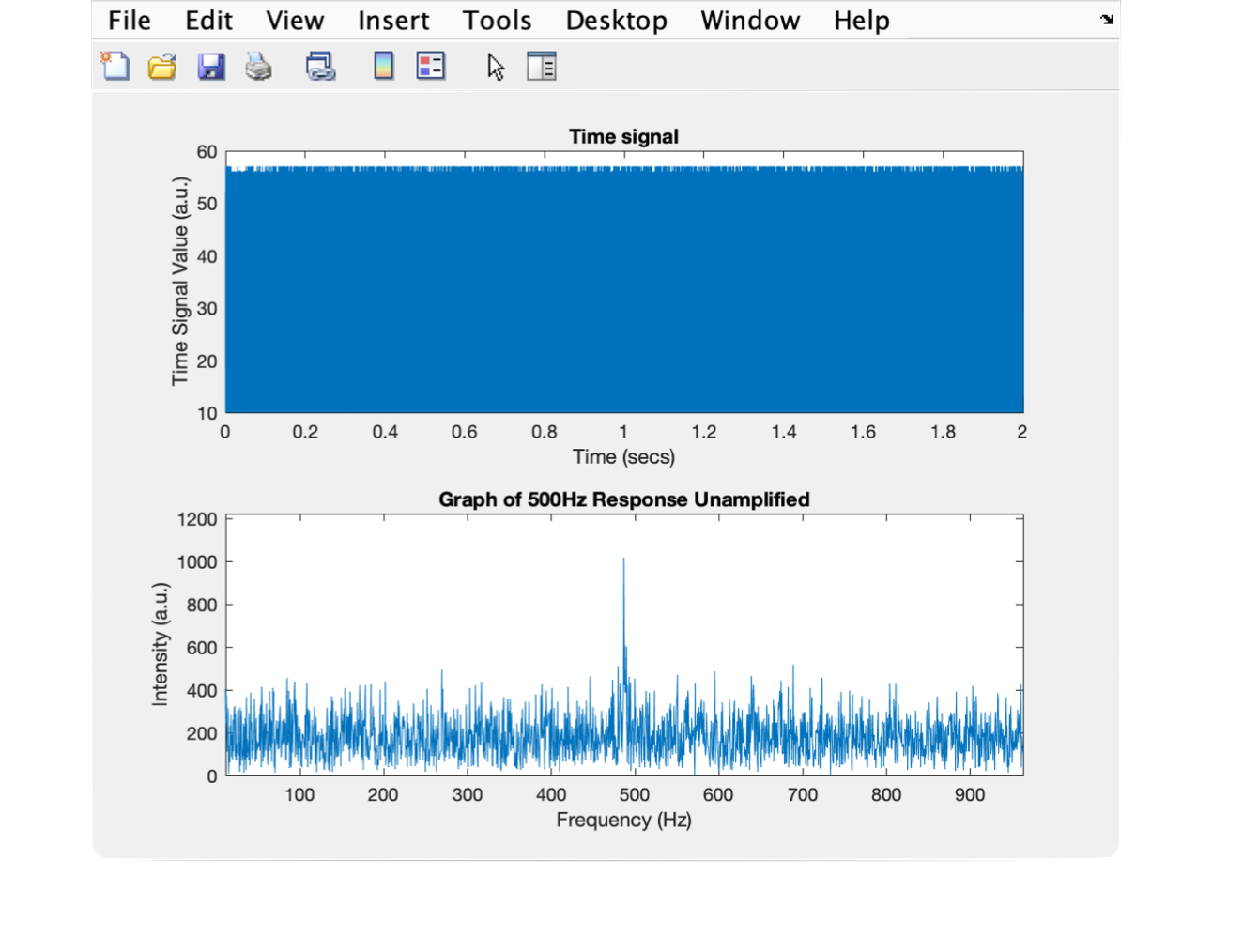This signal is unamplified, which makes the noise to peak ratio fairly large, something that can be improved with the use of an operational amplifier.

### The Amplifier

Next, an amplifier was introduced to our microphone circuit.

Figure 6: The Amplification Circuit Schematic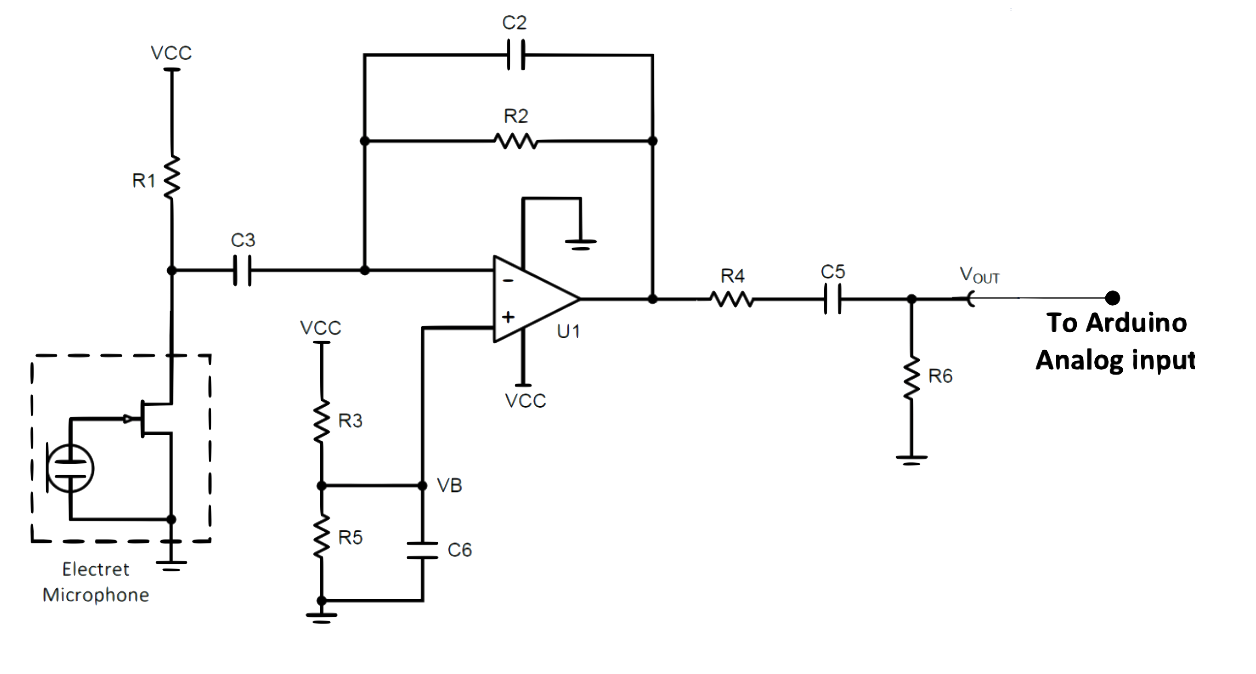The analog pin used on the arduino remained A5, the arduino was not reflashed, and the same MATLAB script was run. However, just by amplifying the signal, the graph produced was drastically different.

Figure 7: The Amplified 500 Hz Response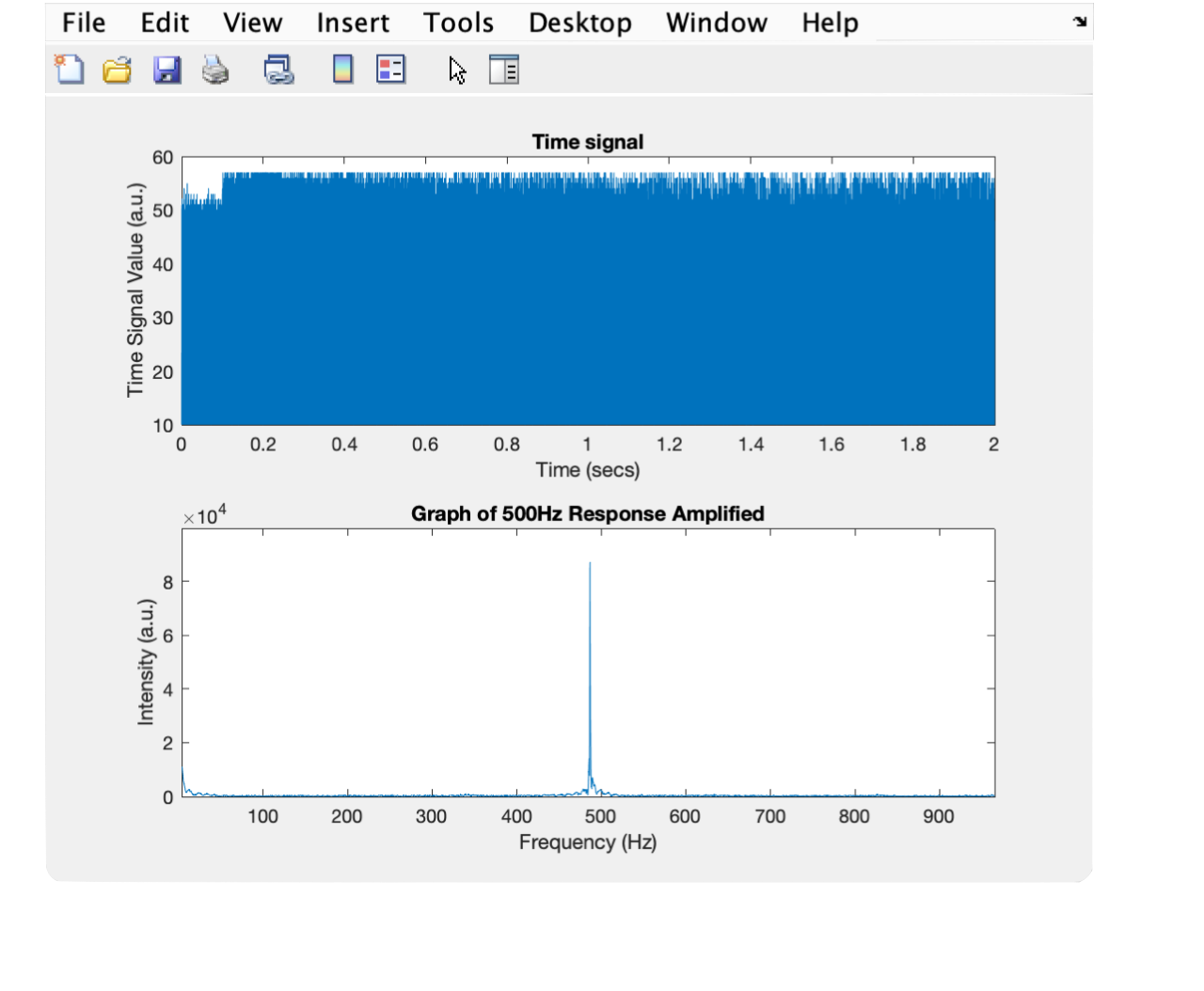Now, since the peak intensity is 10x higher than it was before, the noise at frequncies not equal to 500 Hz is so small in comparison that it can hardly be seen, resulting in a much cleaner looking graph.

### The High Pass Filter

Next, we added a high pass filter to our circuit. The code on the arduino did not change, but the MATLAB script was edited to change the sound played. Instead of a constant 500 Hz signal, we would now sweep from 100Hz to 2000Hz. Picking the high pass filter parameters, with `R = 22 kOhm` and `C = 10 nF`, gets us a cutoff frequency of `723 Hz`. 723 Hz is right inside the working range of our 100-2000 Hz sweep. In order to get the full frequency response H of the filter, we needed to run two sweeps: one with the filter applied (Y) and one without (X). Then, in MATLAB, `H = Y./X`. Then, to put it in decibles like LT Spice, `H_dB = 20*log(H)`. Then, the smoothed H (in decibles) can finally be plotted against frequency.

Figure 8: The Frequency Response of our Filter vs Simulated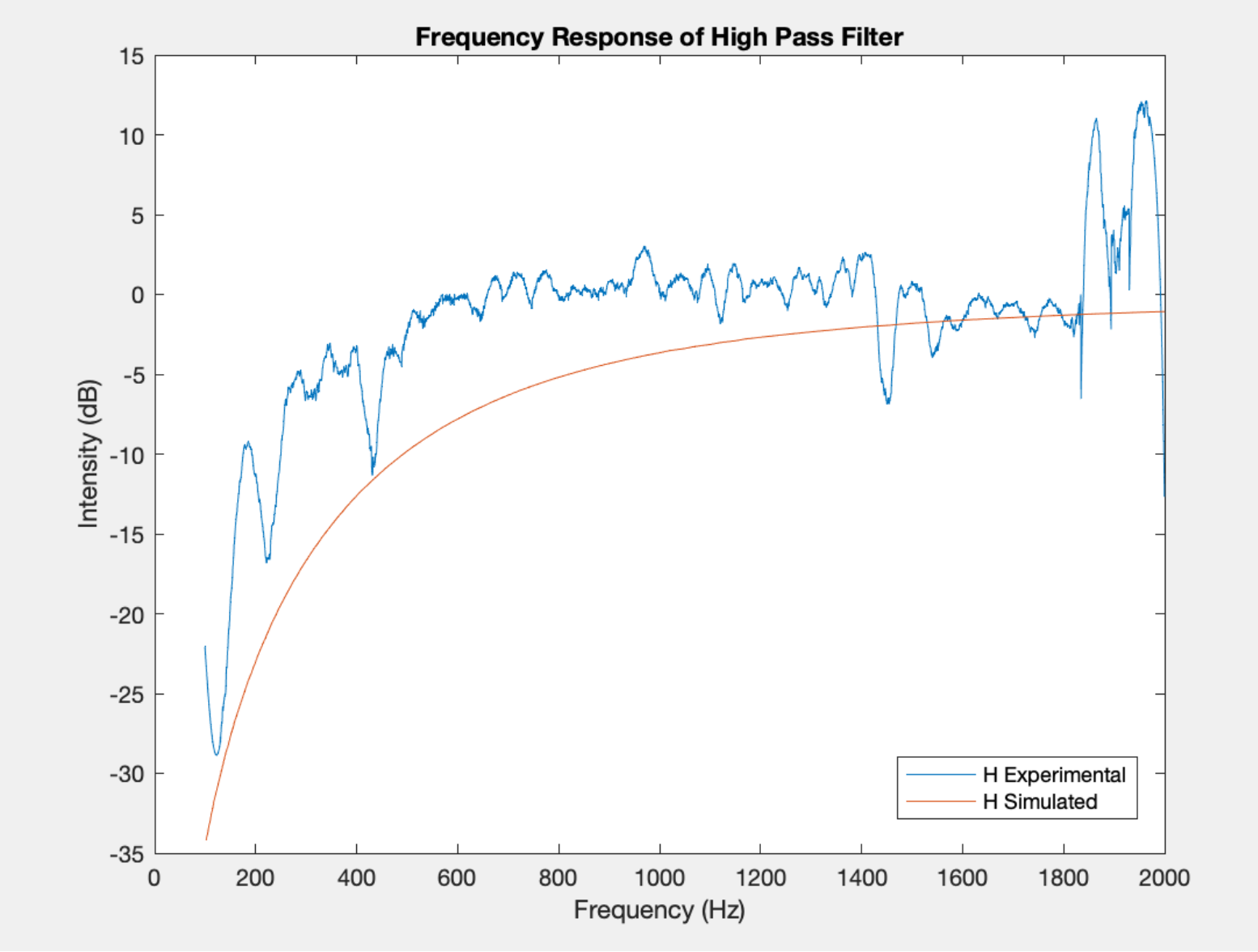The overlay between the high pass filter simulated using LT spice and the one obtained experimentally shows that our filter is indeed behaving as expected although much less perfect and more noisy.

### The FFT

For the final step of part 3, we want to generate FFTs using the arduino. Now, instead of using our ADC Freerun code that has been flashed to the Arduino since the beginning of this part, we must edit it to store FFT values.

Figure 9: The FFT on Serial Monitor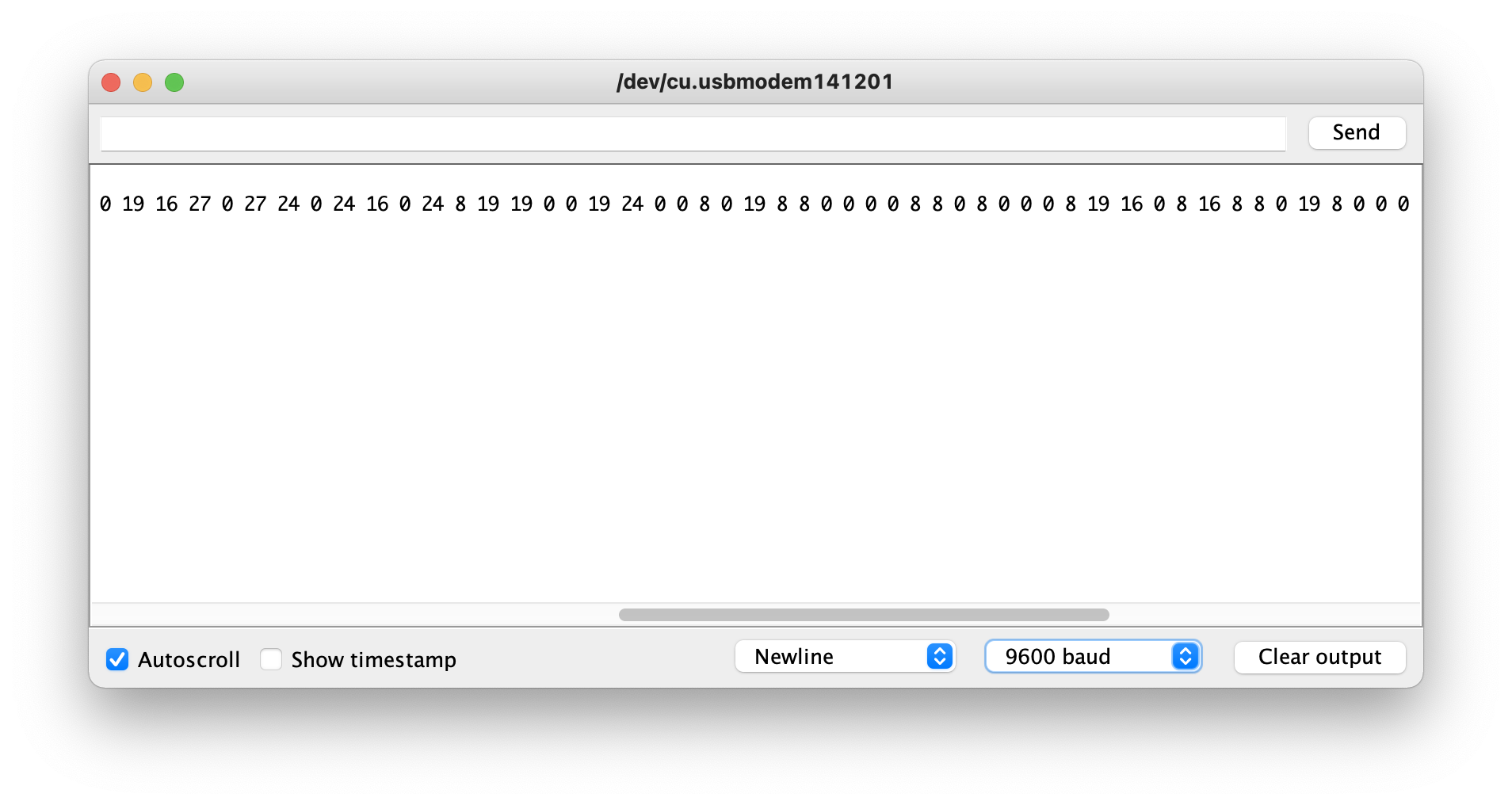In order to graph these results to interpret them visually, we use MATLAB. By assigning the Arduino’s serial output via a copy and paste to a variable, call it `y`, we can run `stem(y)` and generate a beautiful FFT plot of the data.

The interrupts are enabled and samples are collected right when the Arduino boots up, so we must be sure to be playing a signal when the reset button on the Arduino is pressed.

Figure 10: Nano-Generated FFT of 500 Hz Signal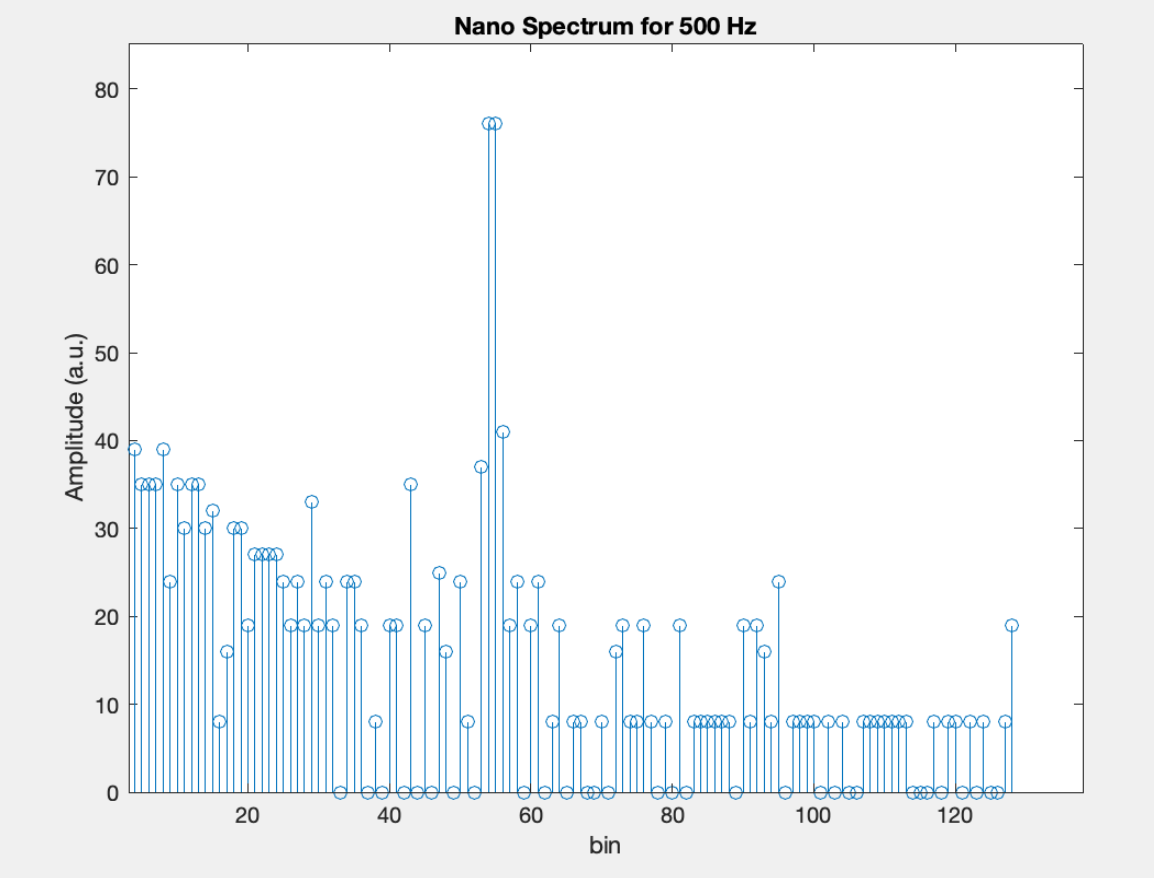Figure 11: Nano-Generated FFT of 700 Hz Signal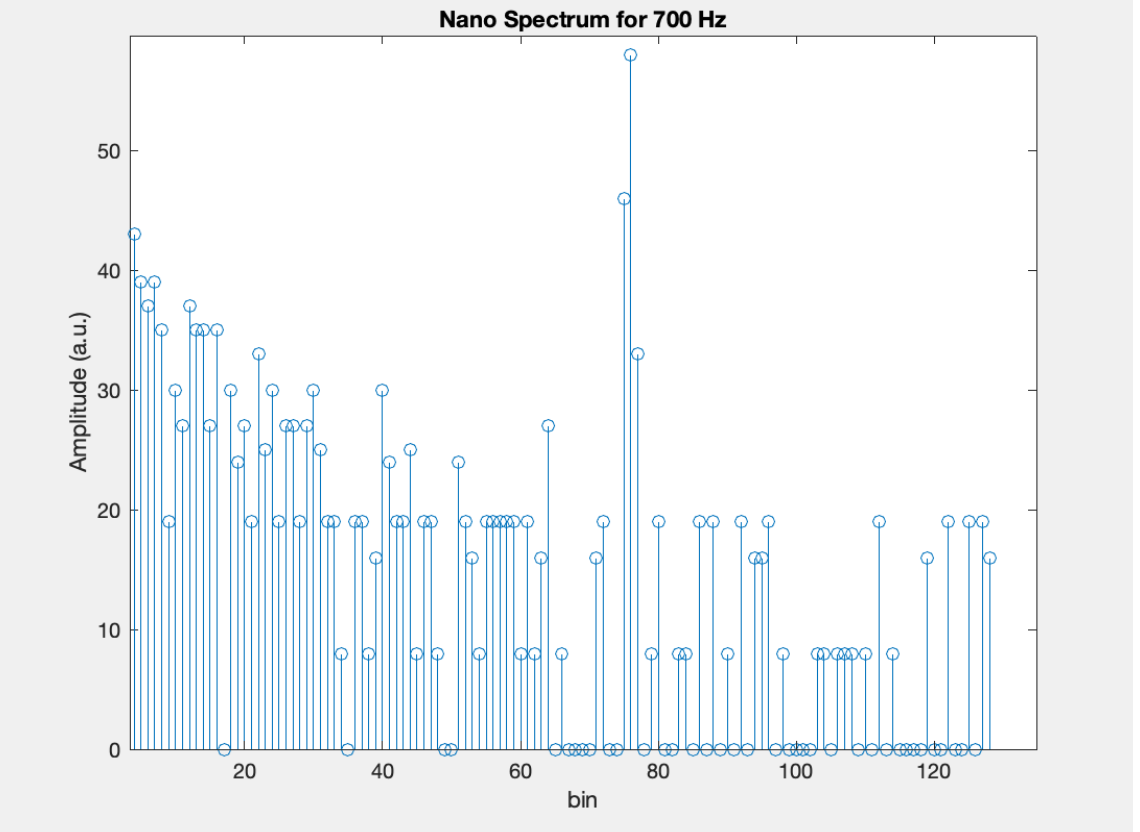Figure 12: Nano-Generated FFT of 900 Hz Signal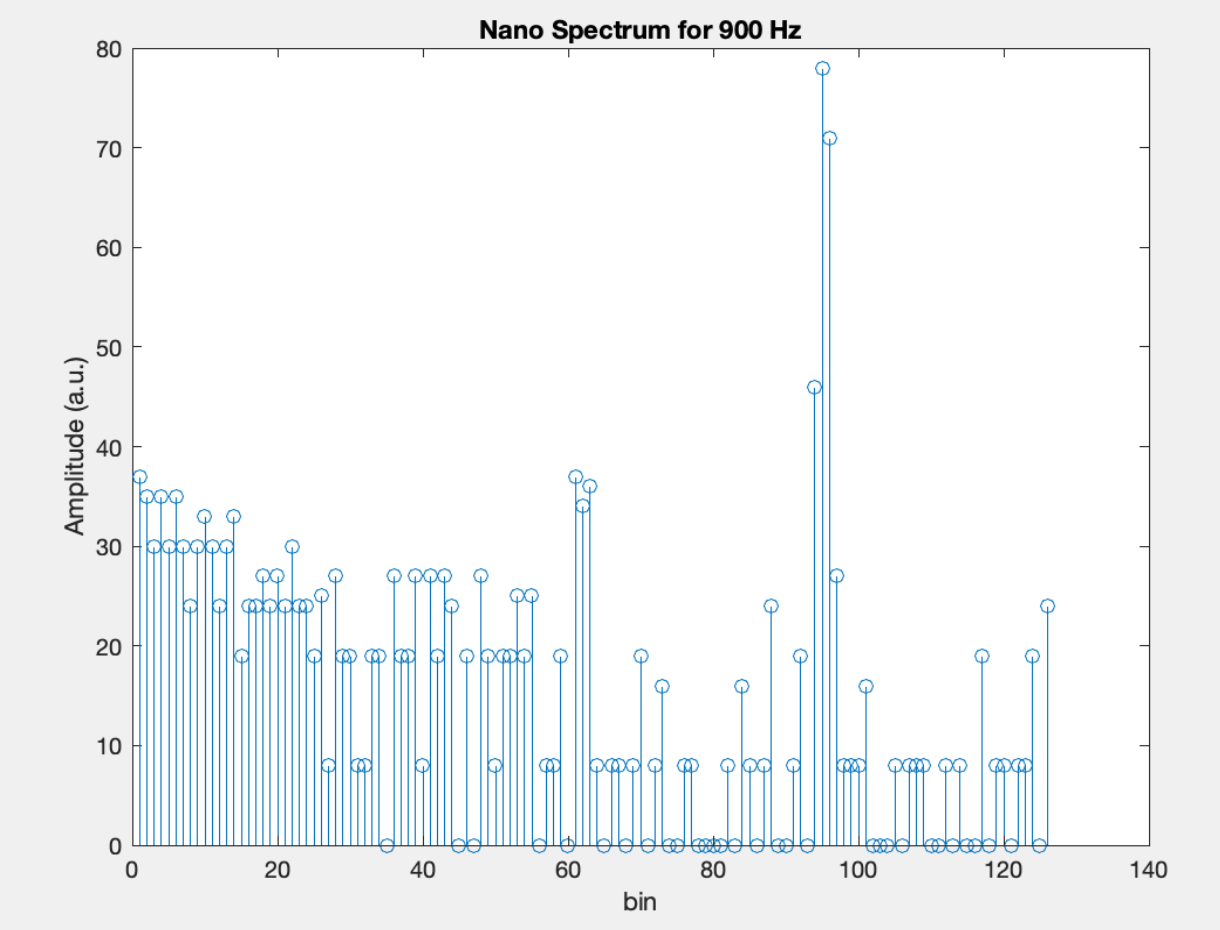### Wrapping Up

Here are a couple photos of our robot with the microphone, the amplifier, and the high pass circuitry all included.